# How to find duplicate values in Excel using Vlookup

You can watch a video tutorial here.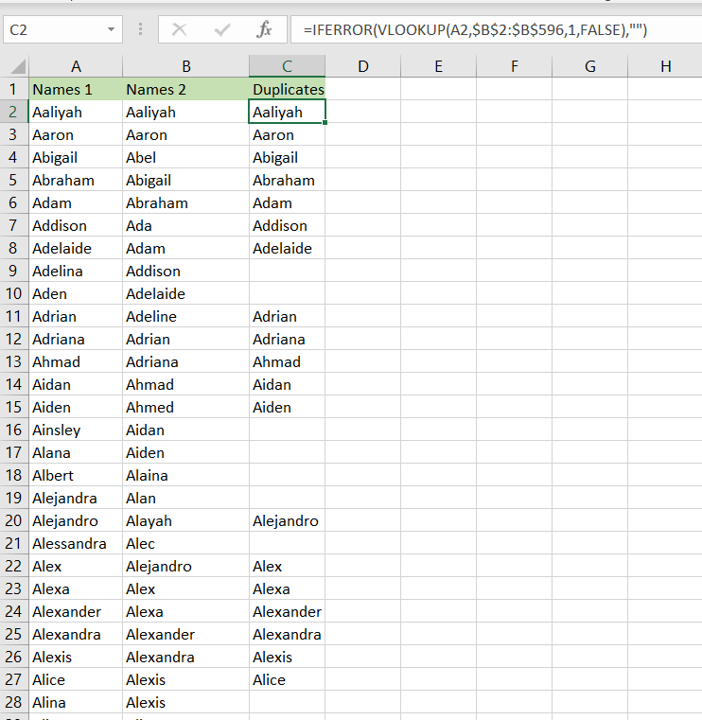Comparing two datasets for duplicates is an operation you will frequently need to do when working with datasets. This happens especially when you are combining data from multiple sources and need to identify duplicates or matches. In Excel, this can be done using Vlookkup:

1. VLOOKUP() function: this searches for a value in the left-most column of a range, and returns the value in the column specified from the row of the match in the range.

a. Syntax: VLOOKUP(lookup_value, table_array, col_index_num, range_lookup)

i. lookup_value: the cell reference of the first value in the key column from Table 1 (Name)
ii. table_array: the range of Table 2 which is made constant with the addition of dollar signs (\$)
iii. col_index_num: the number of the column in Table 2 that has to be retrieved (1 i.e. the Name)
iv. range_lookup: TRUE will return an approximate match, FALSE will return an exact match

### Step 1 – Start typing the formula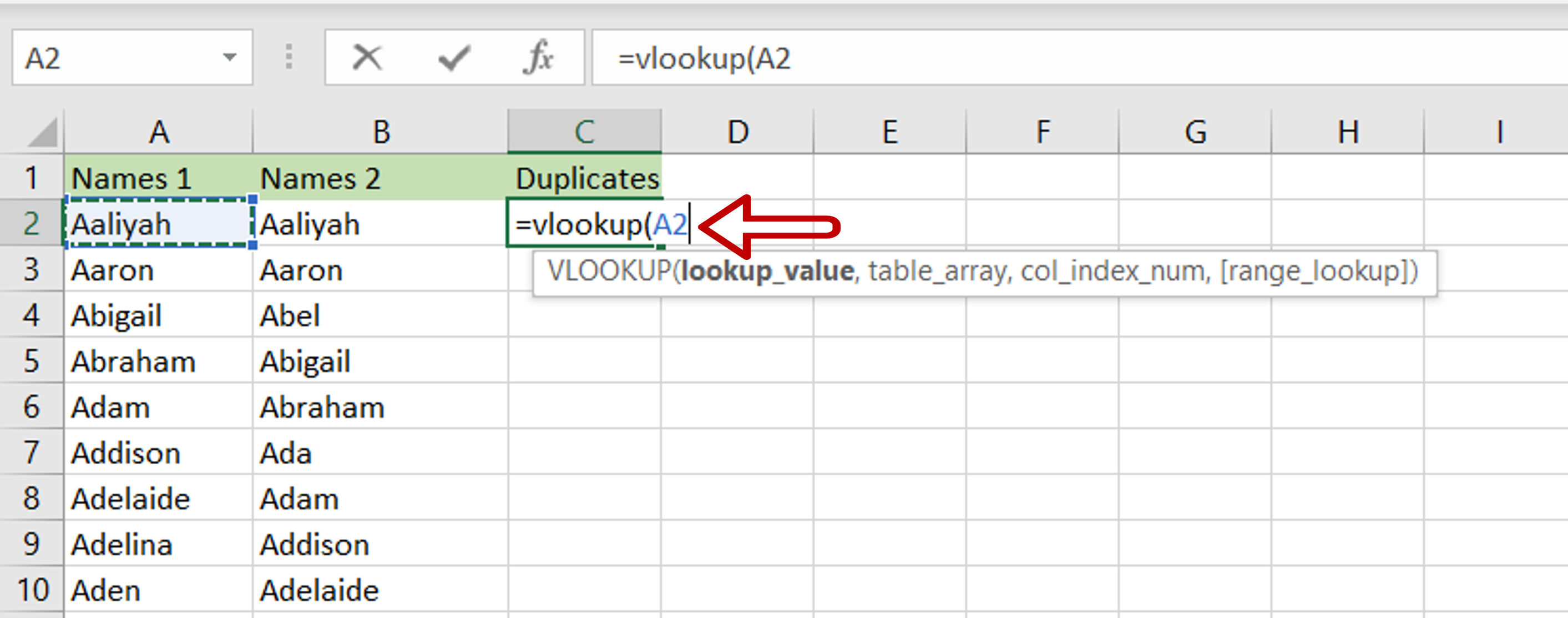– Select the cell where the result is to appear
– Start typing the formula using cell references:
=VLOOKUP(Names 1,

### Step 2 – Reference the second range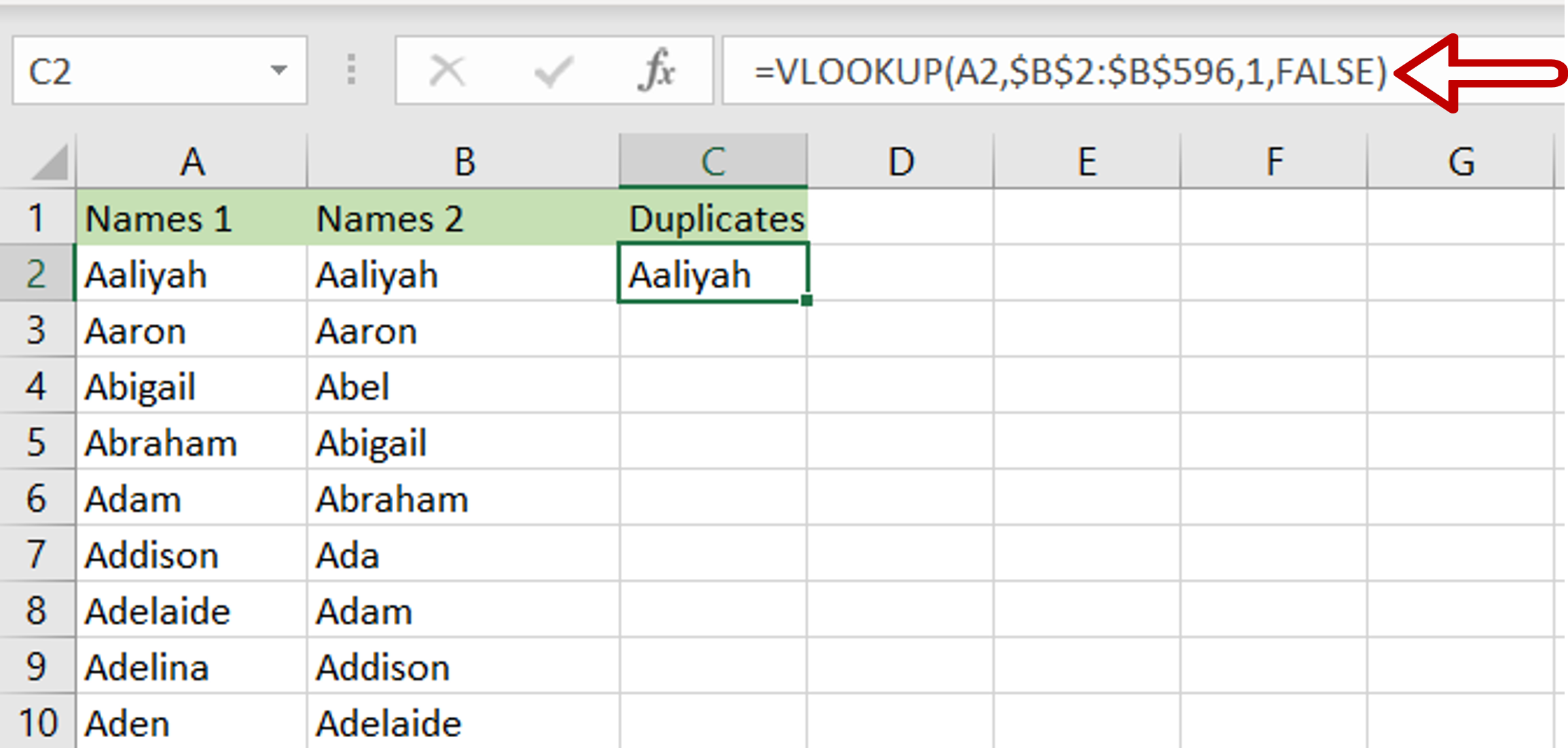– Select the range of the column that is being compared
– The range will be added to the formula
– Complete the formula”
= VLOOKUP(Names 1, \$Names 2\$, 1, FALSE)
– The col_index_num is set to 1 because there is only one column
– The second range is made constant by selecting the cell references and pressing F4
– Press Enter

### Step 3 – Enhance the formula to handle errors i.e. missing values– If the lookup function cannot find a match in the second file i.e. ‘Names 2’, an error message will be returned  – #N/A
– Change the formula to display an empty cell if a match cannot be found:
= IFERROR(<vlookup formula>,””)

### Step 4 – Copy the formula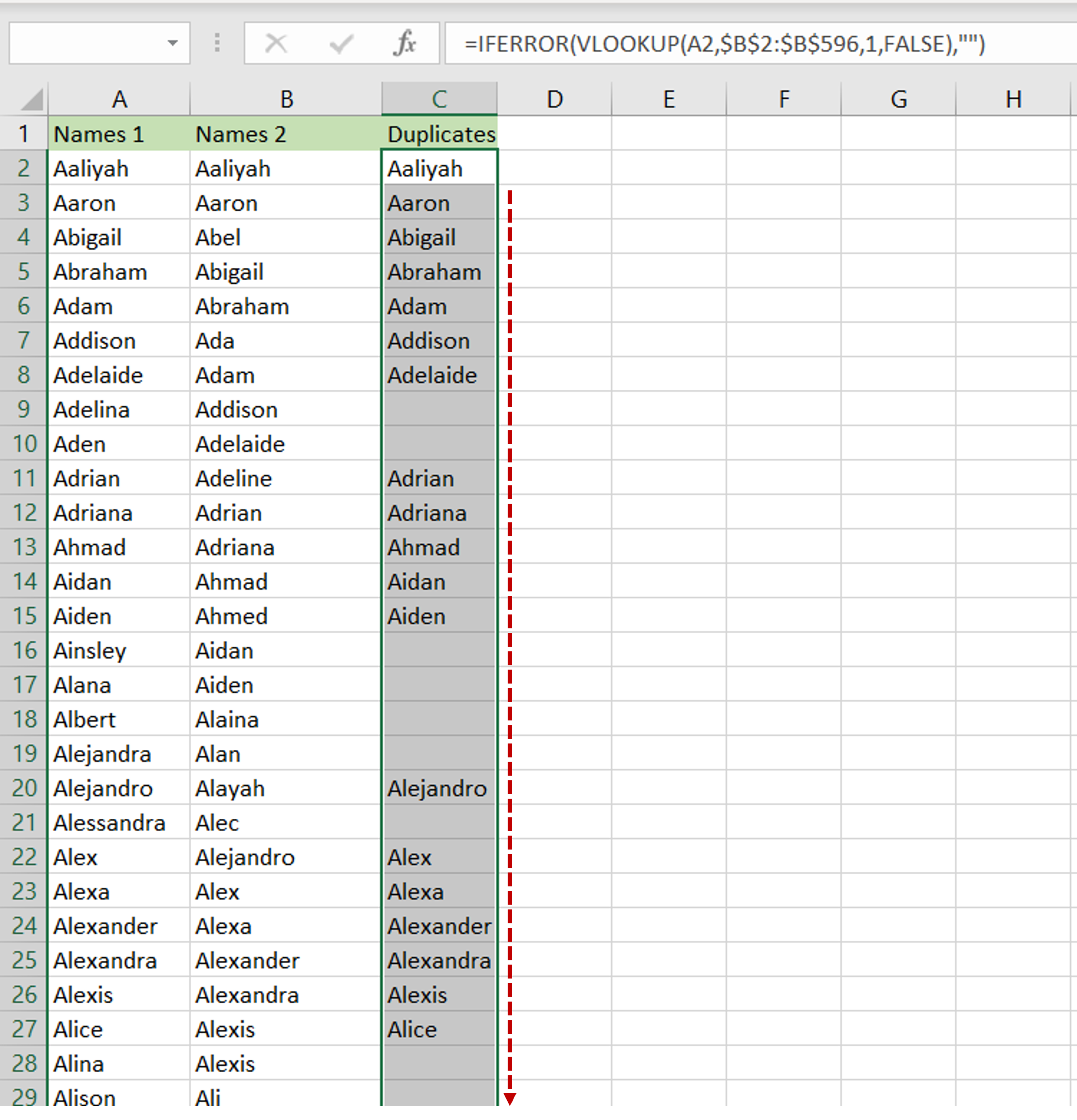– Select the cell with the formula and press Ctrl+C
– Press Ctrl+Shift+Down arrow to go to the end of the column
– Press Enter
– The formula will be copied to the rest of the column

### Step 5 – Check the result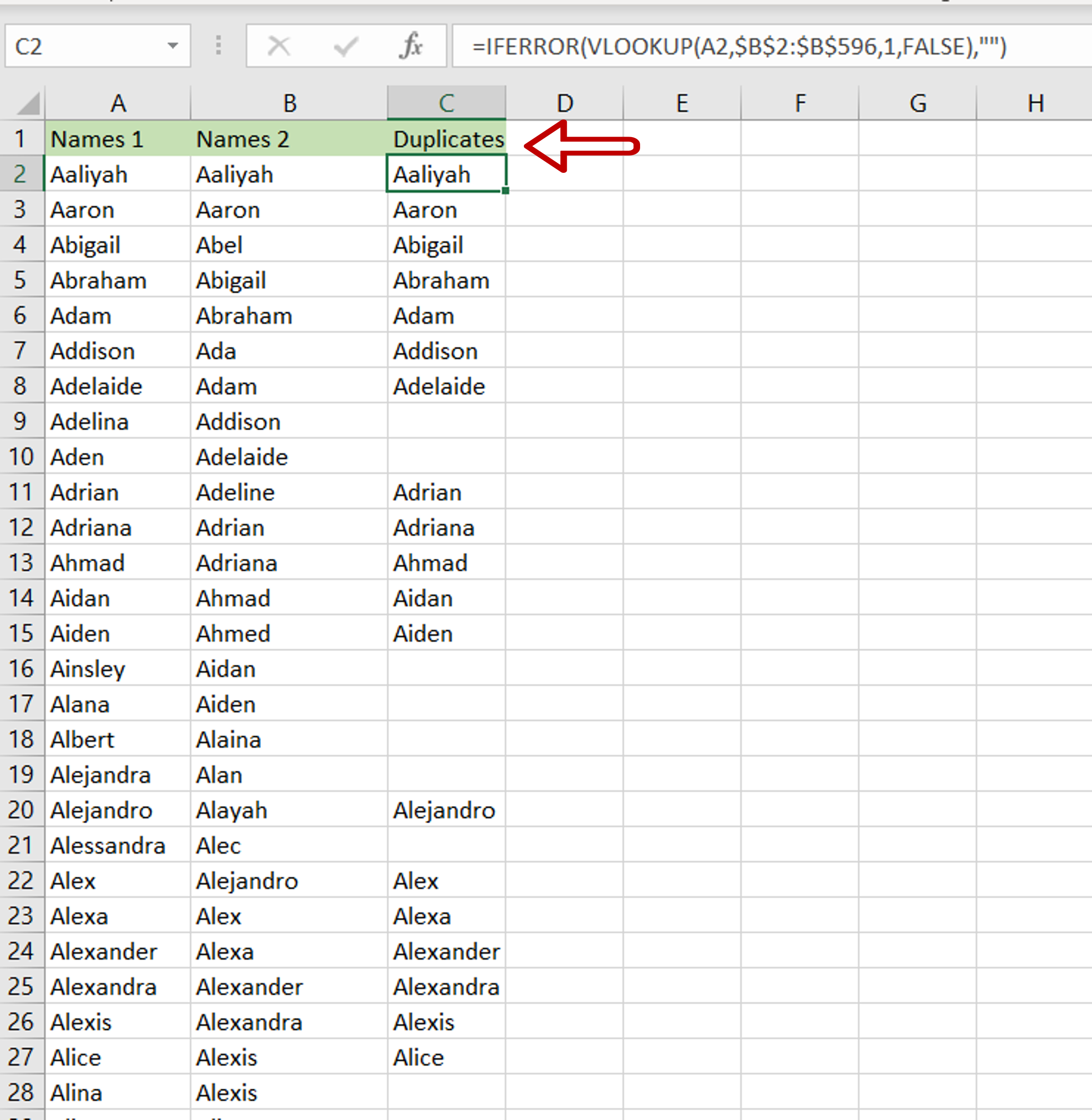– The ‘Duplicates’ column has the list of duplicates between the two columns